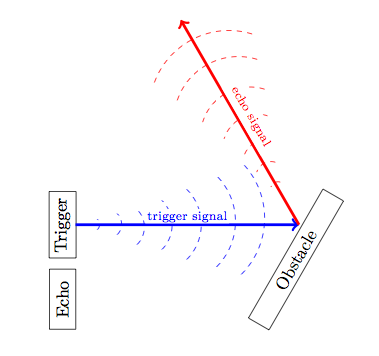### Distance sensor working on a Raspberry Pi

A fun way to start playing with your Raspberry Pi is using LEDs and buttons, from there you can try other sensors. This is a simple, hands on tutorial to get the HC-SR04 distance sensor working using the Raspberry Pi.

Materials

The SensorHC-SR04 Ultrasonic Sensor
The HC-SR04 is a very simple ultrasonic sensor. Though it is not very delicate, it is still advised to manipulate with caution.

Basically, this sensor emits a sound pulse, that echoes on the measured object's surface and is reflected back to the sensor. The time that the pulse took from being emitted to received is the base used to calculate the distance.

Bear in mind that the surface should be parallel to the sensor, if not the reflection and the distance measurement will be affected.Correct way to operateIncorrect way to operate
Pinout:
• Vcc: 5V power supply
• Trig: Trigger pin (input)
• Gnd: Power ground
The way it works is by changing the trigger pin to logic 1 (in this case 3.3V from the Raspberry Pi GPIO will do), this will generate an 8 pulse at 40 kHz. This will be followed by the receiving pin changing to logic 1 (5V) and will thus remain until the pulse is reflected back and changed to logic 0 (0V).

The formula for distance is:

$d=\frac{v_{sound}\times t_{pulse}}{2}$
• d is the distance
• v is the speed of sound, 340 m/s
• t is the time it took the pulse to get back to the sensor
Note: The reason that it is divided by 2 is because the pulse has to travel twice the distance to get back to the source.

The Wiring

As the Raspberry Pi GPIO can only handle 3.3V input voltage, we will need to use a voltage divider. This will divide the 5V from the sensor and will keep the GPIO safe. It is important to note that the resistances values should always be R1 < R2 < 2R1.The wiring with voltage divider

The Software

Copy or download this code and run it in terminal, remember to use root privileges. It should look something like the image below. You can always halt the execution by pressing Ctrl+C at any time.

# Derick DeLeon
# 2014-01
# HC-SR04_example.py
# This is an atempt to make the HC-SR04 ultrasonic distance sensor work

import RPi.GPIO as GPIO
import time

def prepare(GPIO_ECHO, GPIO_TRIGGER):
# Set pins as output and input
GPIO.setup(GPIO_TRIGGER,GPIO.OUT)  # Trigger
GPIO.setup(GPIO_ECHO,GPIO.IN)      # Echo

# Set trigger to False (Low)
GPIO.output(GPIO_TRIGGER, False)

# Allow module to settle
time.sleep(0.5)

# get the distance from the sensor, echo - the input from sensor
def get_distance(GPIO_ECHO, GPIO_TRIGGER):

# Send 10us pulse to trigger
GPIO.output(GPIO_TRIGGER, True)
time.sleep(0.00001)
GPIO.output(GPIO_TRIGGER, False)
start = time.time()

while GPIO.input(GPIO_ECHO)==0:
start = time.time()

while GPIO.input(GPIO_ECHO)==1:
stop = time.time()

# Calculate pulse length
elapsed = stop-start

# Distance pulse travelled in that time is time
# multiplied by the speed of sound (cm/s)
distance = elapsed * 34300

# That was the distance there and back so halve the value
distance = distance / 2
return distance

# MAIN
GPIO.setwarnings(False)
# Set to Board pin number mapping
GPIO.setmode(GPIO.BCM)
# Set up the GPIO channels
trigger = 17
echo = 27
prepare(echo, trigger)
try:
while True:
d = get_distance(echo, trigger)
print d
except KeyboardInterrupt:
GPIO.cleanup()

GPIO.cleanup()


1.1.